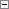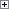Gets the length of a string you define.

Length := STRLEN(String)

Parameters

String

Type: Text constant or Code

The string for which you want to determine the length.Property Value/Return Value

Type: Integer

The length of String.Remarks

The difference between the STRLEN function and the MAXSTRLEN Function (Code, Text) is that the STRLEN returns the actual number of characters in the input string, whereas MAXSTRLEN returns the maximum defined length of the input string.

In Microsoft Dynamics NAV 2013 R2, if you call STRLEN on a Variant, then you get an error that the contents of the parameter are not valid. In earlier versions of Microsoft Dynamics NAV, if you call STRLEN on a Variant, then 0 is returned.Example

This example shows the difference between the STRLEN and the MAXSTRLEN functions.

This example requires that you create the following variables and text constants in the C/AL Globals window.

Variable name DataType Length

City

Text

30

MaxLength

Integer

Not applicable

Length

Integer

Not applicable

Text constant ENU value

Text000

Atlanta

Text001

The MAXSTRLEN function returns: %1,\

Text002

whereas the STRLEN function returns: %2Copy Code
City := Text000;
MaxLength := MAXSTRLEN(City);
Length := STRLEN(City);
MESSAGE(Text001 + Text002, MaxLength, Length);

The message window displays the following:

The MAXSTRLEN function returns: 30

whereas the STRLEN function returns: 7

This shows that the MAXLENGTH function returns the maximum possible length according to the definition of the string variable, whereas STRLEN returns the actual length of the text.

Code Data Type
Text Data Type